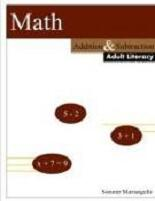SFr. 25.90 € 25.90BTC 0.0009LTC 0.267ETH 0.0158bestellen Artikel-Nr. 21859648 Diesen Artikel in meineWunschliste Diesen Artikel weiterempfehlen Diesen Preis beobachten Weitersagen: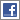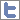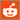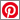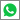Autor(en): Sommer Matriangello
 MATH: Addition And Subtraction(Buch)
Dieser Artikel gilt, aufgrund seiner Grösse, beim Versand als 2 Artikel!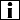Übersicht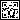Auf mobile öffnenLieferstatus: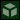i.d.R. innert 14-24 Tagen versandfertig Genre: Psychologie / Pädagogik ISBN: 9781329736450 EAN-Code: 9781329736450 Verlag: Lulu.com Einband: Kartoniert Sprache: English Dimensionen: H 280 mm / B 210 mm / D 7 mm Gewicht: 348 gr Seiten: 134 Zus. Info: Paperback Bewertung: Titel bewerten / Meinung schreiben
 Inhalt: Math: Addition and Subtraction book is excellent for adults who need to learn math from scratch. It starts with very simple 1 + 1 addition and moves on to math equations like if x + 5 = 15, what is x? The book is easy to follow and makes teaching an adult learner easy and simple.At the completion of this book the learner will be able to: . determine number place values . round numbers . add single digit numbers . add double digit numbers without carry-over . add double digit numbers with carry-over . add three and four digit numbers with carry-over . subtract single digit numbers . subtract double digit numbers without borrowing . subtract double digit numbers with borrowing . subtract three digit numbers with borrowing . subtract numbers with zeros . basic addition and subtraction equations . using rounding to estimate answers

 Empfehlungen...Basic Lessons For Babies & Toddlers 2: Basic Math - (DVD - Code 1) Foundation Maths 7th Edition plus MyLab Math with - (Buch) USA Las Matemáticas: Descríbelo (Use Math: Describ - (Buch) Introductory & Intermediate Algebra with Integrate - (Buch) Game of Nim and Graph Theory: Fun, Hands-On Activi - (Buch) Math Made Easy: Fifth Grade - (Buch) Geometry and Topology: Fun, Hands-On Activities fo - (Buch) USA Las Matemáticas: Compáralo (Use Math: Compare - (Buch) Flunk: Chemistry And Math - (Musik CD) Graph Paper Notebook: Quad Ruled Graph Paper Compo - (Buch) Weitersuchen in   DVD/FILME   CDS   GAMES   BÜCHERN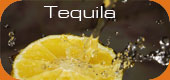Wird aktuell angeschaut... SFr. 20.50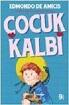Buch SFr. 47.90Buch SFr. 8.50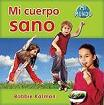Buch SFr. 35.90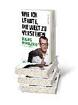Buch SFr. 29.50Buch SFr. 21.90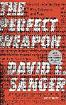Buch SFr. 41.50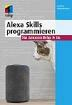Buch SFr. 20.50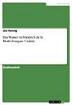Buch

AGB | Datenschutzerklärung | Mein Konto | Impressum | Partnerprogramm
Newsletter |Newsfeed |Facebook |Twitter
Forbidden Planet AG © 1999-2021
Alle Angaben ohne Gewähr
 SUCHEN Alle Sprachen Deutsch Englisch Spanisch Französisch

 Kategorien Im Sortiment stöbern Genres Hörbücher Aktionen Infos Mein Konto Warenkorb Meine Wunschliste Kundenservice Recherchedienst Fragen / AGB / Kontakt Partnerprogramm Impressum
 © by Forbidden Planet AG 1999-2021